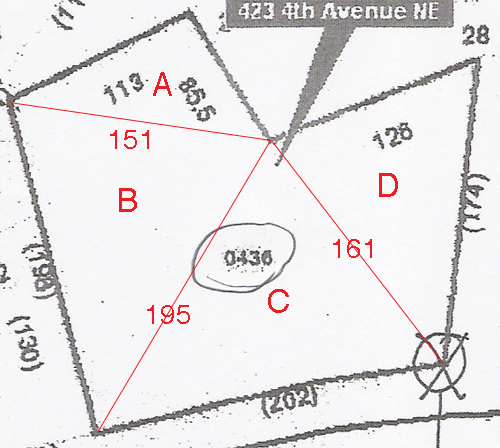SEARCH HOMEMath Central Quandaries & QueriesI need the attached land parcel (#0436) converted to acreage. This would be of great help to me. Thanks, RickyRicky,

I can make an estimate based on the diagram you sent. I added some lines and labels.My estimate is based on two assumptions, that the diagram is drawn to scale and that the measurements are in feet. Starting at the lower right corner of the parcel (at the X) and going around the parcel counterclockwise I read the dimensions as 174 ft, 128 ft, 85.5 ft, 113, ft 198 ft and 202 ft.

I subdivided the parcel into four triangles, A, B, C and D, using the four red lines in the diagram and then from the inferred scale I found the lengths of these four lines, 161 ft, 151 ft and 195 ft. I did this because the area of a triangle can be calculated from the lengths of its sides using Heron's Formula. Using the dimensions in the diagram and Heron's Formula I found

Triangle Area
A 4782.6 sq ft
B 13694.3 sq ft
C 14592.4 sq ft
D 9855.4 sq ft
Total 42924.7 sq ft

One acres is 43560 square feet so 42924.7 sq ft is 42924.7/43560 = 0.99 acres.

Again I remind you that this is only an estimate based on the diagram you sent,
PennyMath Central is supported by the University of Regina and The Pacific Institute for the Mathematical Sciences.